# Selina Solutions Concise Mathematics Class 6 Chapter 5: Natural And Whole Numbers Exercise 5(C)

Selina Solutions Concise Mathematics Class 6 Chapter 5 Natural Numbers And Whole Numbers Exercise 5(C) contains exercise wise problems on closure property, commutative law of multiplication and distributive law of multiplication. Regular practice of Selina Solutions improves students’ knowledge about the concepts discussed here. It will help students to solve tricky questions with ease that would appear in the final examination. For further details, students can take the help of Selina Solutions solved by the faculty at BYJU’S. Avail Selina Solutions Concise Mathematics Class 6 Chapter 5 Natural Numbers And Whole Numbers Exercise 5(C), PDF for free download from the links given below

## Selina Solutions Concise Mathematics Class 6 Chapter 5: Natural Numbers And Whole Numbers Exercise 5(C) Download PDF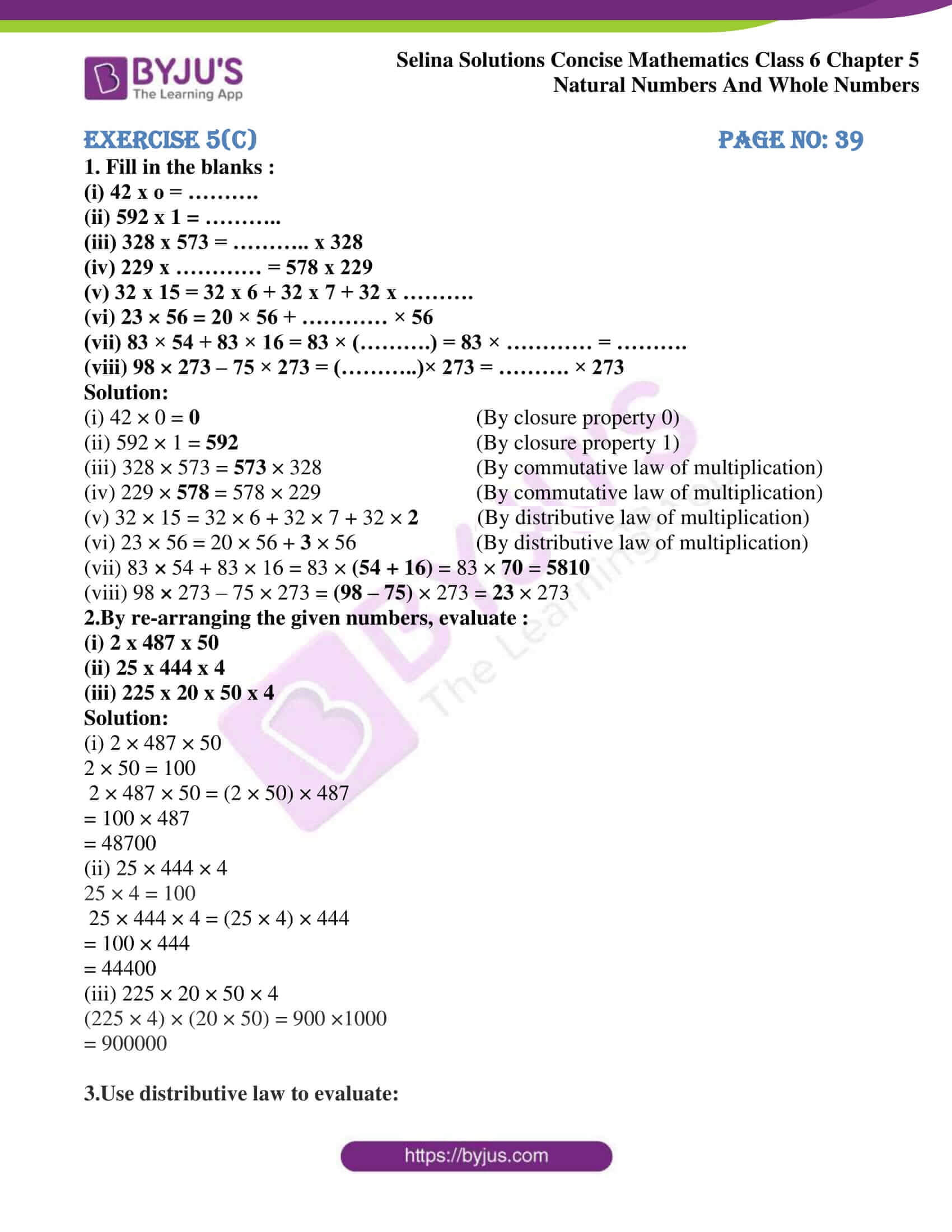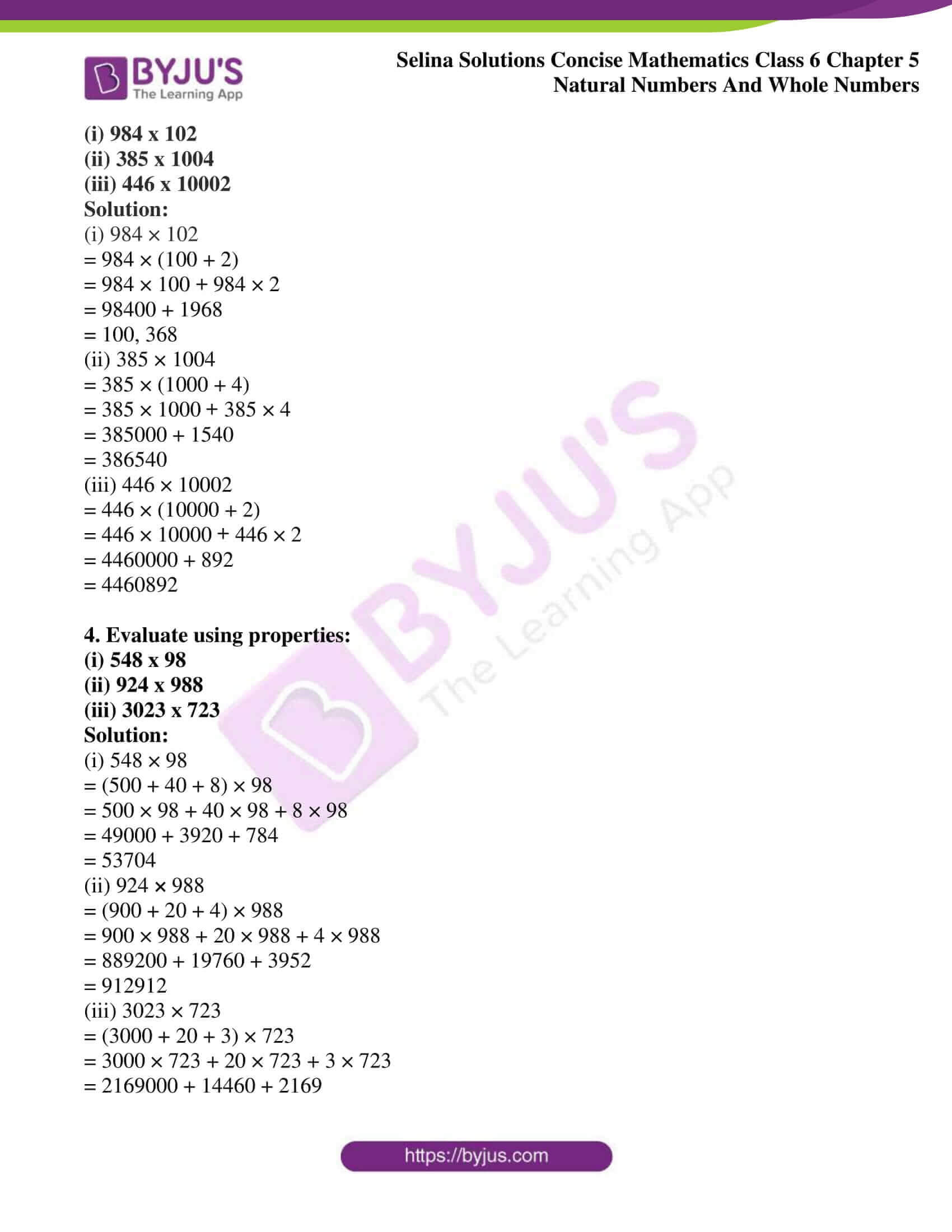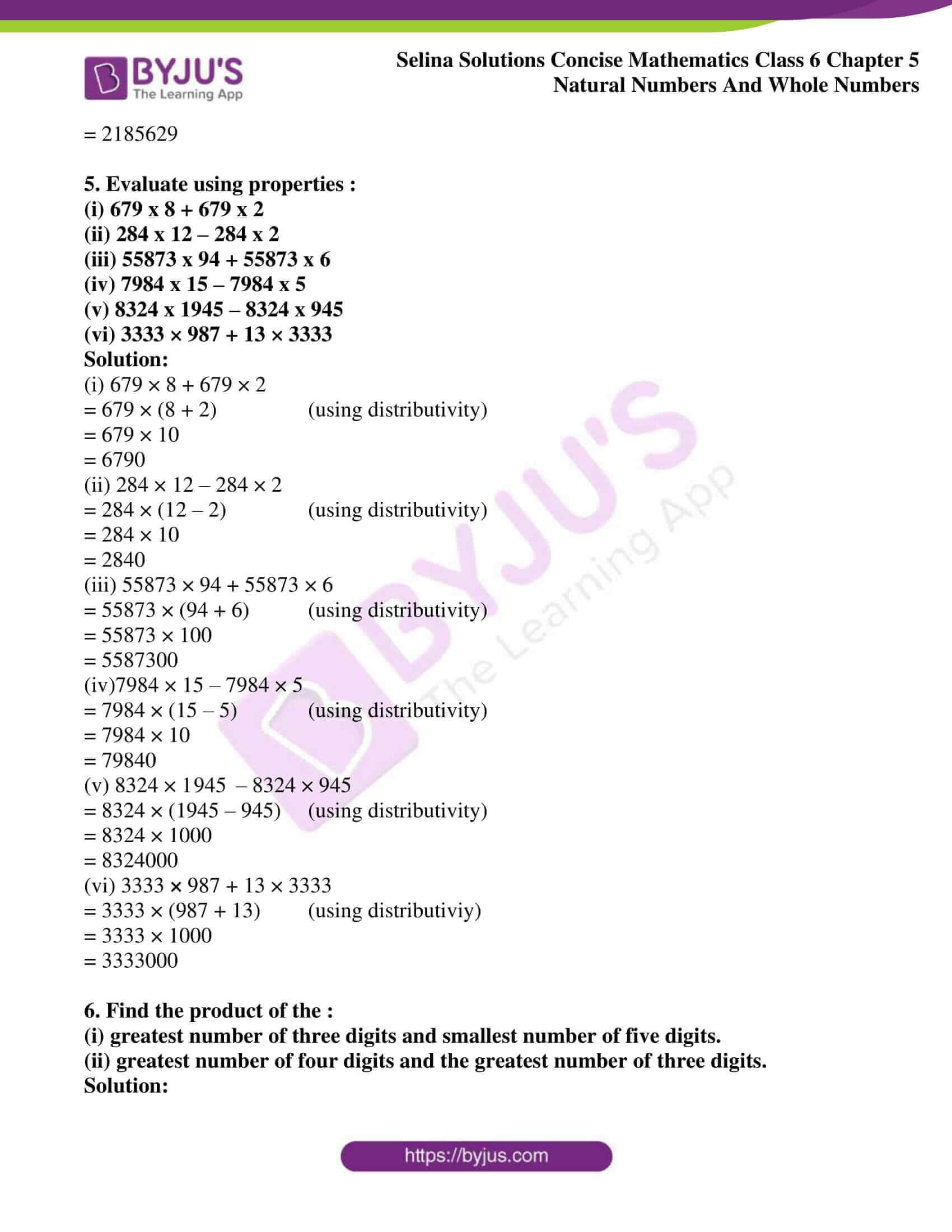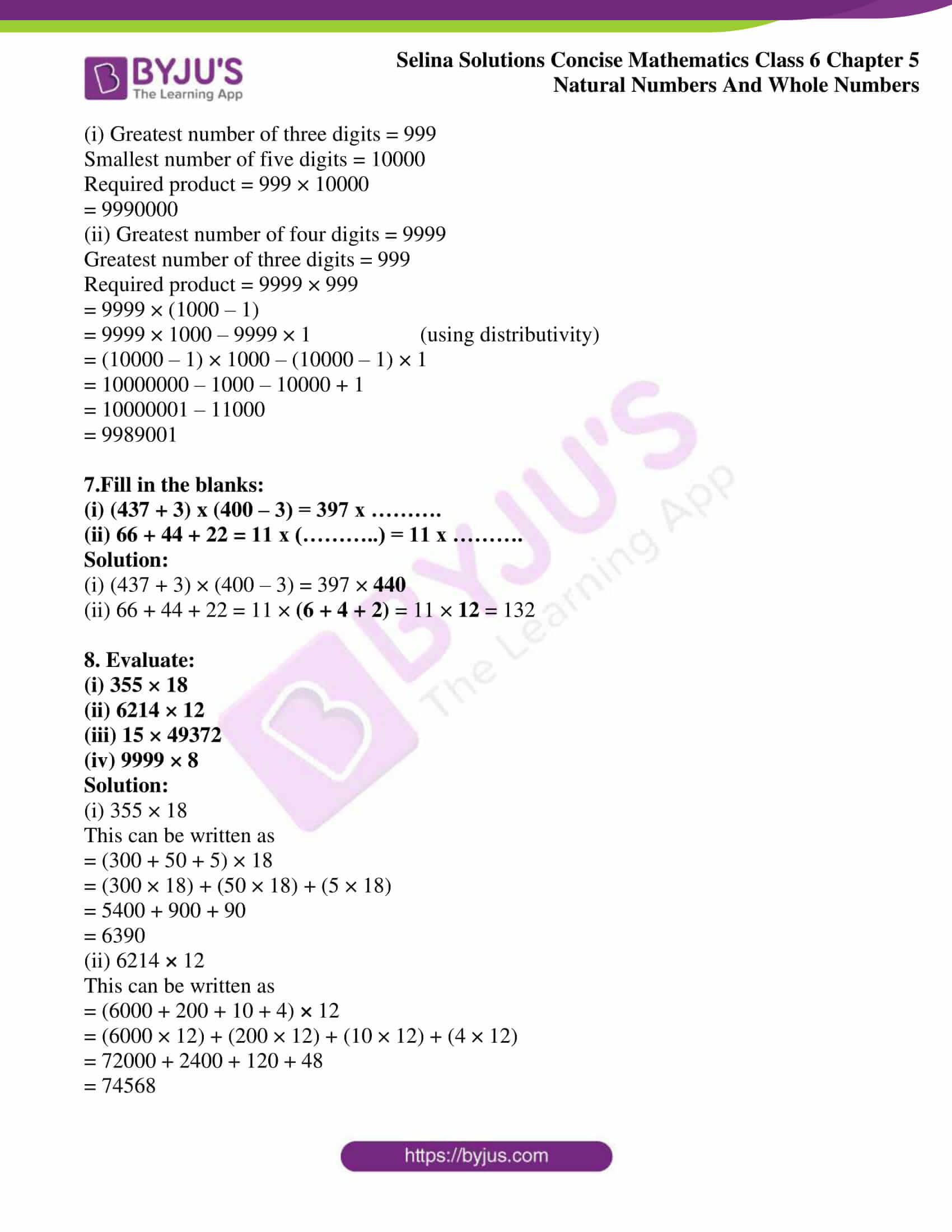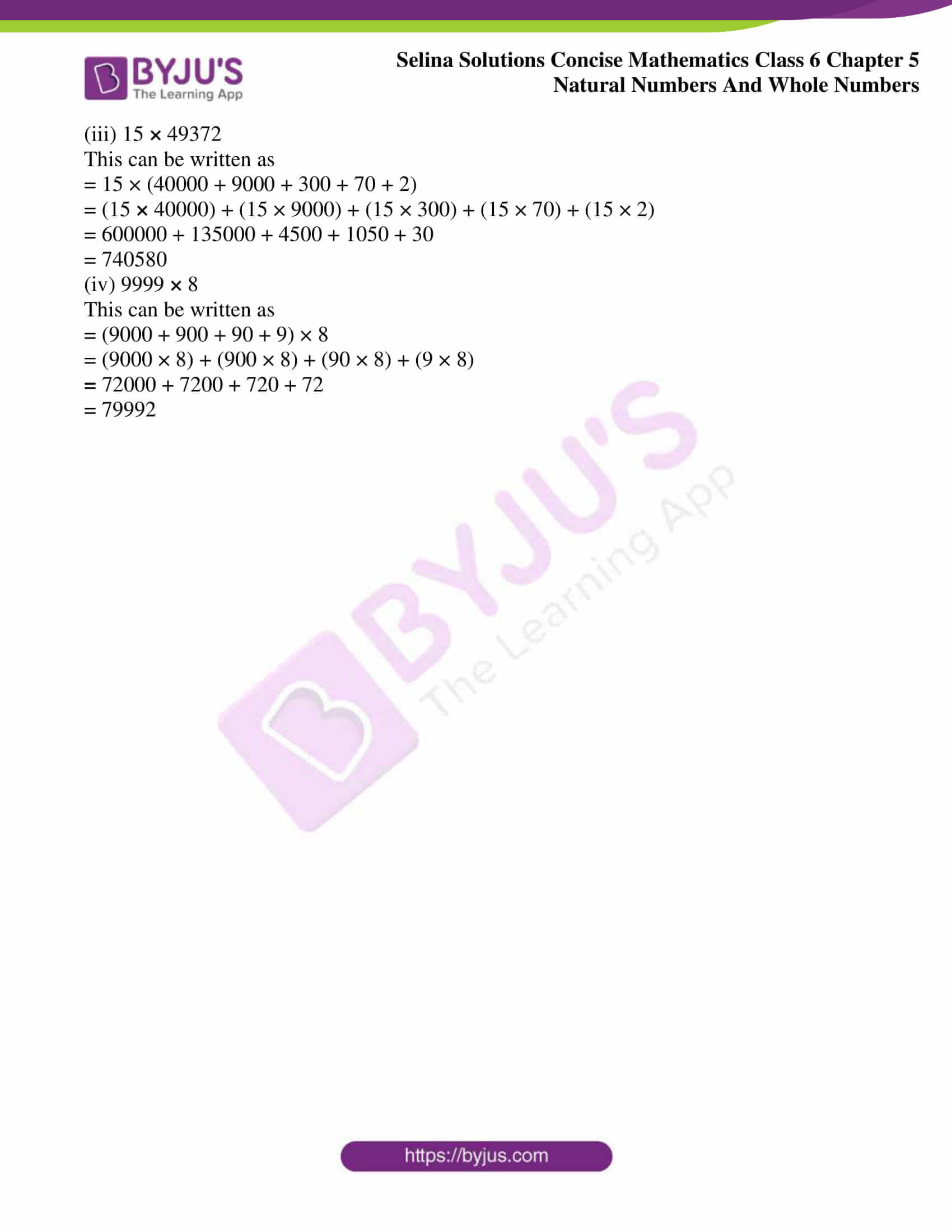### Access other exercises of Selina Solutions Concise Mathematics Class 6 Chapter 5: Natural Numbers And Whole Numbers

Exercise 5(A) Solutions

Exercise 5(B) Solutions

Exercise 5(D) Solutions

Exercise 5(E) Solutions

Exercise 5(F) Solutions

### Access Selina Solutions Concise Mathematics Class 6 Chapter 5: Natural Numbers And Whole Numbers Exercise 5(C)

#### Exercise 5(C) page no: 39

1. Fill in the blanks :
(i) 42 x o = ……….
(ii) 592 x 1 = ………..
(iii) 328 x 573 = ……….. x 328
(iv) 229 x ………… = 578 x 229
(v) 32 x 15 = 32 x 6 + 32 x 7 + 32 x ……….

(vi) 23 × 56 = 20 × 56 + ………… × 56

(vii) 83 × 54 + 83 × 16 = 83 × (……….) = 83 × ………… = ……….

(viii) 98 × 273 – 75 × 273 = (………..)× 273 = ………. × 273

Solution:

(i) 42 × 0 = 0 (By closure property 0)

(ii) 592 × 1 = 592 (By closure property 1)

(iii) 328 × 573 = 573 × 328 (By commutative law of multiplication)

(iv) 229 × 578 = 578 × 229 (By commutative law of multiplication)

(v) 32 × 15 = 32 × 6 + 32 × 7 + 32 × 2 (By distributive law of multiplication)

(vi) 23 × 56 = 20 × 56 + 3 × 56 (By distributive law of multiplication)

(vii) 83 × 54 + 83 × 16 = 83 × (54 + 16) = 83 × 70 = 5810

(viii) 98 × 273 – 75 × 273 = (98 – 75) × 273 = 23 × 273

2.By re-arranging the given numbers, evaluate :
(i) 2 x 487 x 50
(ii) 25 x 444 x 4
(iii) 225 x 20 x 50 x 4

Solution:

(i) 2 × 487 × 50

2 × 50 = 100

2 × 487 × 50 = (2 × 50) × 487

= 100 × 487

= 48700

(ii) 25 × 444 × 4

25 × 4 = 100

25 × 444 × 4 = (25 × 4) × 444

= 100 × 444

= 44400

(iii) 225 × 20 × 50 × 4

(225 × 4) × (20 × 50) = 900 ×1000

= 900000

3.Use distributive law to evaluate:
(i) 984 x 102
(ii) 385 x 1004
(iii) 446 x 10002

Solution:

(i) 984 × 102

= 984 × (100 + 2)

= 984 × 100 + 984 × 2

= 98400 + 1968

= 100, 368

(ii) 385 × 1004

= 385 × (1000 + 4)

= 385 × 1000 + 385 × 4

= 385000 + 1540

= 386540

(iii) 446 × 10002

= 446 × (10000 + 2)

= 446 × 10000 + 446 × 2

= 4460000 + 892

= 4460892

4. Evaluate using properties:
(i) 548 x 98
(ii) 924 x 988
(iii) 3023 x 723

Solution:

(i) 548 × 98

= (500 + 40 + 8) × 98

= 500 × 98 + 40 × 98 + 8 × 98

= 49000 + 3920 + 784

= 53704

(ii) 924 × 988

= (900 + 20 + 4) × 988

= 900 × 988 + 20 × 988 + 4 × 988

= 889200 + 19760 + 3952

= 912912

(iii) 3023 × 723

= (3000 + 20 + 3) × 723

= 3000 × 723 + 20 × 723 + 3 × 723

= 2169000 + 14460 + 2169

= 2185629

5. Evaluate using properties :
(i) 679 x 8 + 679 x 2
(ii) 284 x 12 – 284 x 2
(iii) 55873 x 94 + 55873 x 6
(iv) 7984 x 15 – 7984 x 5
(v) 8324 x 1945 – 8324 x 945

(vi) 3333 × 987 + 13 × 3333

Solution:

(i) 679 × 8 + 679 × 2

= 679 × (8 + 2) (using distributivity)

= 679 × 10

= 6790

(ii) 284 × 12 – 284 × 2

= 284 × (12 – 2) (using distributivity)

= 284 × 10

= 2840

(iii) 55873 × 94 + 55873 × 6

= 55873 × (94 + 6) (using distributivity)

= 55873 × 100

= 5587300

(iv)7984 × 15 – 7984 × 5

= 7984 × (15 – 5) (using distributivity)

= 7984 × 10

= 79840

(v) 8324 × 1945 – 8324 × 945

= 8324 × (1945 – 945) (using distributivity)

= 8324 × 1000

= 8324000

(vi) 3333 × 987 + 13 × 3333

= 3333 × (987 + 13) (using distributiviy)

= 3333 × 1000

= 3333000

6. Find the product of the :
(i) greatest number of three digits and smallest number of five digits.
(ii) greatest number of four digits and the greatest number of three digits.

Solution:

(i) Greatest number of three digits = 999

Smallest number of five digits = 10000

Required product = 999 × 10000

= 9990000

(ii) Greatest number of four digits = 9999

Greatest number of three digits = 999

Required product = 9999 × 999

= 9999 × (1000 – 1)

= 9999 × 1000 – 9999 × 1 (using distributivity)

= (10000 – 1) × 1000 – (10000 – 1) × 1

= 10000000 – 1000 – 10000 + 1

= 10000001 – 11000

= 9989001

7.Fill in the blanks:
(i) (437 + 3) x (400 – 3) = 397 x ……….
(ii) 66 + 44 + 22 = 11 x (………..) = 11 x ……….

Solution:

(i) (437 + 3) × (400 – 3) = 397 × 440

(ii) 66 + 44 + 22 = 11 × (6 + 4 + 2) = 11 × 12 = 132

8. Evaluate:

(i) 355 × 18

(ii) 6214 × 12

(iii) 15 × 49372

(iv) 9999 × 8

Solution:

(i) 355 × 18

This can be written as

= (300 + 50 + 5) × 18

= (300 × 18) + (50 × 18) + (5 × 18)

= 5400 + 900 + 90

= 6390

(ii) 6214 × 12

This can be written as

= (6000 + 200 + 10 + 4) × 12

= (6000 × 12) + (200 × 12) + (10 × 12) + (4 × 12)

= 72000 + 2400 + 120 + 48

= 74568

(iii) 15 × 49372

This can be written as

= 15 × (40000 + 9000 + 300 + 70 + 2)

= (15 × 40000) + (15 × 9000) + (15 × 300) + (15 × 70) + (15 × 2)

= 600000 + 135000 + 4500 + 1050 + 30

= 740580

(iv) 9999 × 8

This can be written as

= (9000 + 900 + 90 + 9) × 8

= (9000 × 8) + (900 × 8) + (90 × 8) + (9 × 8)

= 72000 + 7200 + 720 + 72

= 79992